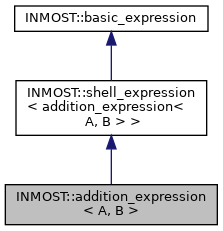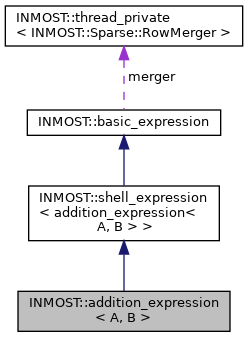INMOST A toolkit for distributed mathematical modeling
INMOST::addition_expression< A, B > Class Template Reference
Inheritance diagram for INMOST::addition_expression< A, B >:[legend]
Collaboration diagram for INMOST::addition_expression< A, B >:[legend]

## Public Member Functions

addition_expression (const shell_expression< A > &pleft, const shell_expression< B > &pright)

__INLINE INMOST_DATA_REAL_TYPE GetValue () const

__INLINE void GetJacobian (INMOST_DATA_REAL_TYPE mult, Sparse::RowMerger &r) const

__INLINE void GetJacobian (INMOST_DATA_REAL_TYPE mult, Sparse::Row &r) const

__INLINE void GetHessian (INMOST_DATA_REAL_TYPE multJ, Sparse::Row &J, INMOST_DATA_REAL_TYPE multH, Sparse::HessianRow &H) const

__INLINE INMOST_DATA_ENUM_TYPE GetCount () constPublic Member Functions inherited from INMOST::shell_expression< addition_expression< A, B > >
operator addition_expression< A, B > & ()

operator const addition_expression< A, B > & () constStatic Protected Attributes inherited from INMOST::basic_expression